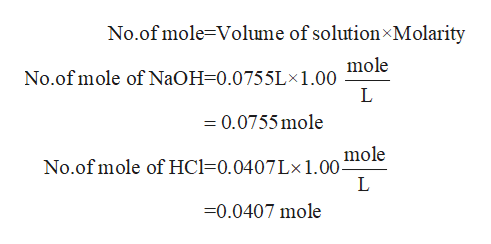# Find the enthalpy of neutralization of 75.5mL 1.00M aqueous sodium hydroxide with 40.7mL 1.00M hydrochloric acid if after mixing the two solutions the temperature went up 6.2∘C. Report the amount of the limiting reagent in mmol: (enthalpies given: NaOH = -425.61, Cl- = -167.16, H+ = 0)

Question
1 views

Find the enthalpy of neutralization of 75.5mL 1.00M aqueous sodium hydroxide with 40.7mL 1.00M hydrochloric acid if after mixing the two solutions the temperature went up 6.2∘C. Report the amount of the limiting reagent in mmol:

(enthalpies given: NaOH = -425.61, Cl- = -167.16, H+ = 0)

check_circle

Step 1

Given that,

Volume of NaOH = 75.5 mL

Molarity of NaOH = 1.00 M

Volume of HCl = 40.7 mL

Molarity of HCl = 1.00 M

Step 2

Now calculate the no.of mole of NaOHhelp_outlineImage TranscriptioncloseNo.of mole-Volume of solution Molarity mole No.of mole of N2OH=0.0755L*1.00 L =0.0755mole mole No.of mole of HCl 0.0407L* 1.00- L 0.0407 mole fullscreen
Step 3

The reactio...

### Want to see the full answer?

See Solution

#### Want to see this answer and more?

Solutions are written by subject experts who are available 24/7. Questions are typically answered within 1 hour.*

See Solution
*Response times may vary by subject and question.
Tagged in

### Quantitative analysis